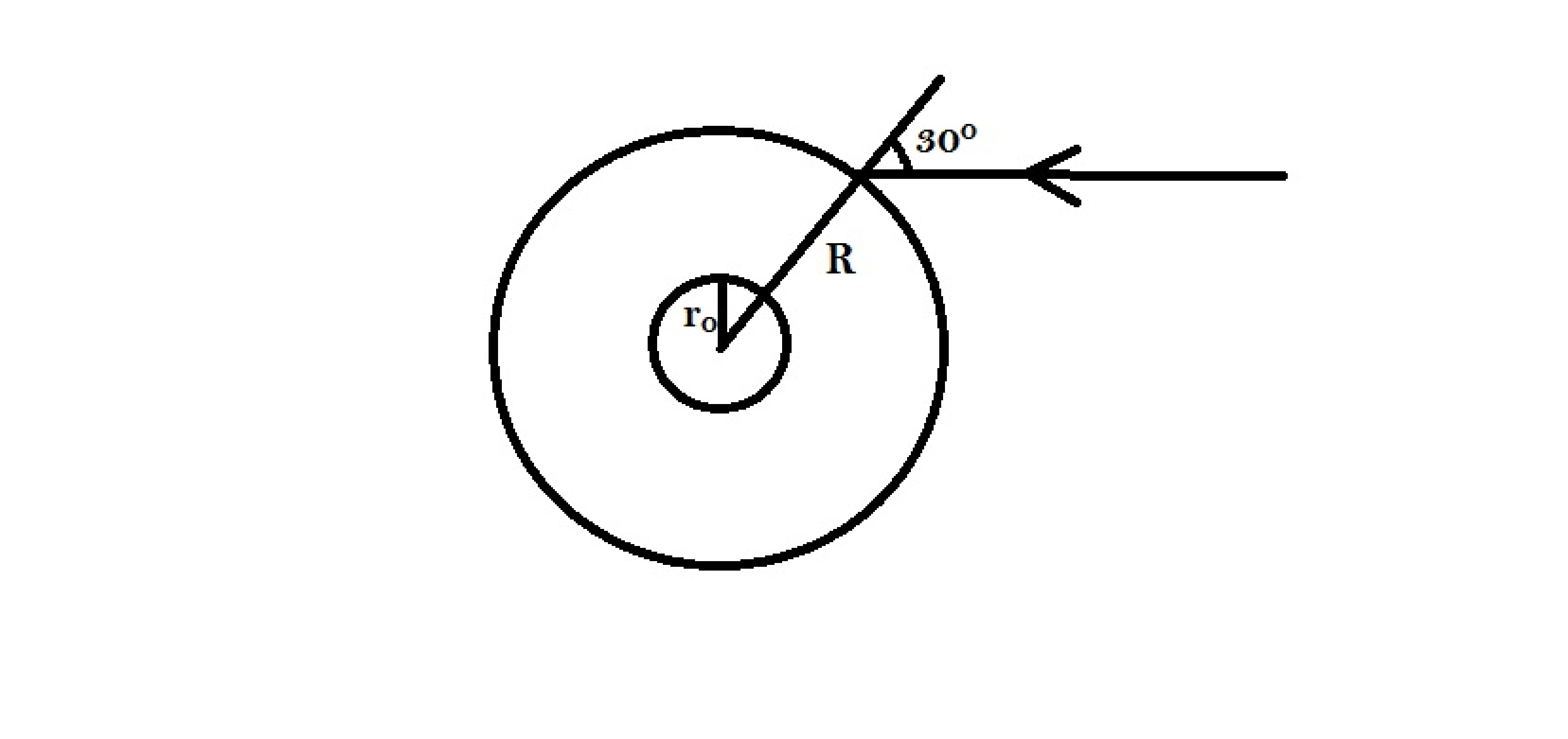# Parallel slabs? I think not!A laser beam propagates through a region whose refractive index varies as $\mu = \mu_0 (\frac{r}{r_0})$. At a distance of R the beam makes an angle of 30 degrees with the normal.

Find the minimum separation between the beam and the center of this region(the point where $r=0$) in cm.

Assume $\mu_0= 1$, $r_0= 3 \text{cm}$, $R= 8\sqrt2\text{ cm}$.

×

Problem Loading...

Note Loading...

Set Loading...